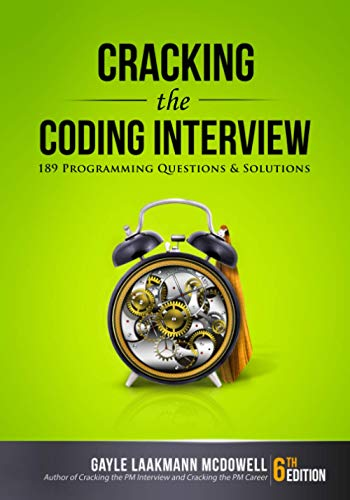# Permutations of a String

Question: Print all the permutations of a string. Write code in C.

Answer: Here is a recursive solution to print all the permutations of a string. However, this solution does not take care of duplicates. It is assumed that there are no duplicates in the string. I left out the implementation of the swap method since that implementation is not important here.

The idea is to keep the first character constant and generate permutations with the rest. Then keep the first two characters constant and generate permutations with the rest until you are out of characters 🙂

```void permutate( char[] str, int index )
{
int i = 0;
if( index == strlen(str) )
{ // We have a permutation so print it
printf(str);
return;
}
for( i = index; i < strlen(str); i++ )
{
swap( str[index], str[i] ); // It doesn't matter how you swap.
permutate( str, index + 1 );
swap( str[index], str[i] );
}
}```
`permutate( "abcdefgh", 0 );`

Now what do we do if there are duplicates in the string? The trick is to sort the characters in the alphabetical order first. We can then ignore the duplicates easily when generate the permutation.

```void permutate( char[] str, int index )
{
int i = 0;
static lastChar = 0;
if( index == strlen(str) )
{ // We have a permutation so print it
printf(str);
return;
}
// Permutate once without any swaps.
permutate( str, index + 1 );
lastChar = str[index];
for( i = index + 1; i < strlen(str); i++ )
{
if( lastChar == str[i] ) {
continue;
} else {
lastChar = str[i];
}
swap( str[index], str[i] ); // It doesn't matter how you swap.
permutate( str, index + 1 );
swap( str[index], str[i] );
}
}```
`permutate( sort("Hello World"), 0 );`

Simple right? Any comments or suggestions are always welcome.

Like this post? Please Digg it or Stumble it.

If you're looking for some serious preparation for your interviews, I'd recommend this book written by a lead Google interviewer. It has 189 programming questions and solutions:## 9 Responses

1.Nick says:

Nevermind, every time I try to do a vector of strings, it removes the word string within LT/GT because it thinks it’s a tag. I give up.

2.Nick says:

Gah, I can’t edit my previous attempt at a post. Apparently the code tag didn’t work. Also, usage should say:
vector anagrams = getAnagrams(“poop”);

3.Nick says:

I did a bit of messing around with Andy Dremeaux’s code below and wrote a C++ version using STL vectors that removes duplicates. (His code does create duplicate anagrams if the word has duplicate characters.)
``` void getAnagramsSimple(string s, string pre, vector &anagrams) { if (s.length() > 0) { for (int i = 0; i < s.length(); ++i) { getAnagramsSimple(s.substr(0, i) + s.substr(i + 1), pre + s[i], anagrams); } } else { anagrams.push_back(pre); } }```

``` ```

```vector getAnagrams(string s) { vector anagrams; getAnagramsSimple(s, "", anagrams); sort(anagrams.begin(), anagrams.end()); anagrams.erase(unique(anagrams.begin(), anagrams.end()), anagrams.end()); return anagrams; } `````` It can be called like this: `````` vector anagrams = getAnagrams("poop"); ```

4.Nick says:

I did a bit of messing around with Andy Dremeaux’s code below and wrote a C++ version using STL vectors that removes duplicates. (His code does create duplicate anagrams if the word has duplicate characters.)
``` void getAnagramsSimple(string s, string pre, vector &anagrams) { if (s.length() > 0) { for (int i = 0; i < s.length(); ++i) { getAnagramsSimple(s.substr(0, i) + s.substr(i + 1), pre + s[i], anagrams); } } else { anagrams.push_back(pre); } }```

``` ```

```vector getAnagrams(string s) { vector anagrams; getAnagramsSimple(s, "", anagrams); sort(anagrams.begin(), anagrams.end()); anagrams.erase(unique(anagrams.begin(), anagrams.end()), anagrams.end()); return anagrams; } ```
It can be called like this:
``` vector anagrams = getAnagrams("poop"); ```

5.Jerry says:

sorry, I meant just “char lastChar = 0;”

6.Jerry says:

I don’t think its correct to put “static lastChar = 0;” to detect duplicates.

I guess just “static char lastChar = 0;” would do the trick.

7.Bansal says:

In case of duplicates the function doesn’t produce some results.
e.g for string “aaaa” the string “aaa” itself is not generated.

Also the assumption that all duplicate characters are clubbed together (because of initial sorting) doesn’t hold while permuting.

A simple solution would be to keep track of all characters used at a stack call while permuting.

void permutate( char[] str, int index )
{
if( index == strlen(str) )
{ // We have a permutation so print it
printf(str);
return;
}

char used;
bzero(used, sizeof(used));

int i;
for( i = index; i < strlen(str); i++ )
{
if( (int)used[arr[i]] != 0)
{
continue;
}

used[arr[i]] = 1;

swap( str[index], str[i] ); // It doesn't matter how you swap.
permutate( str, index + 1 );
swap( str[index], str[i] );
}
}

8.LeFunk says:

Nah, the latter function creates duplicates, if some characters repeat in the word – try “poop” for example.

9.Andy Dremeaux says:

Blech. Far too complex for a simple problem. Here’s a simpler and easier to understand solution in ActionScript 3 (nothing fancy) that yields no duplicates.

``` function p(s:String, pre:String):void { if (s.length < 1) trace(pre); else for (var i:int = 0; i < s.length; i++) p(s.substr(0,i) + s.substr(i+1), pre + s.charAt(i)); }```

``` ```

```p("abcd", ""); ```

XHTML: These are some of the tags you can use: `<a href=""> <b> <blockquote> <code> <em> <i> <strike> <strong>`